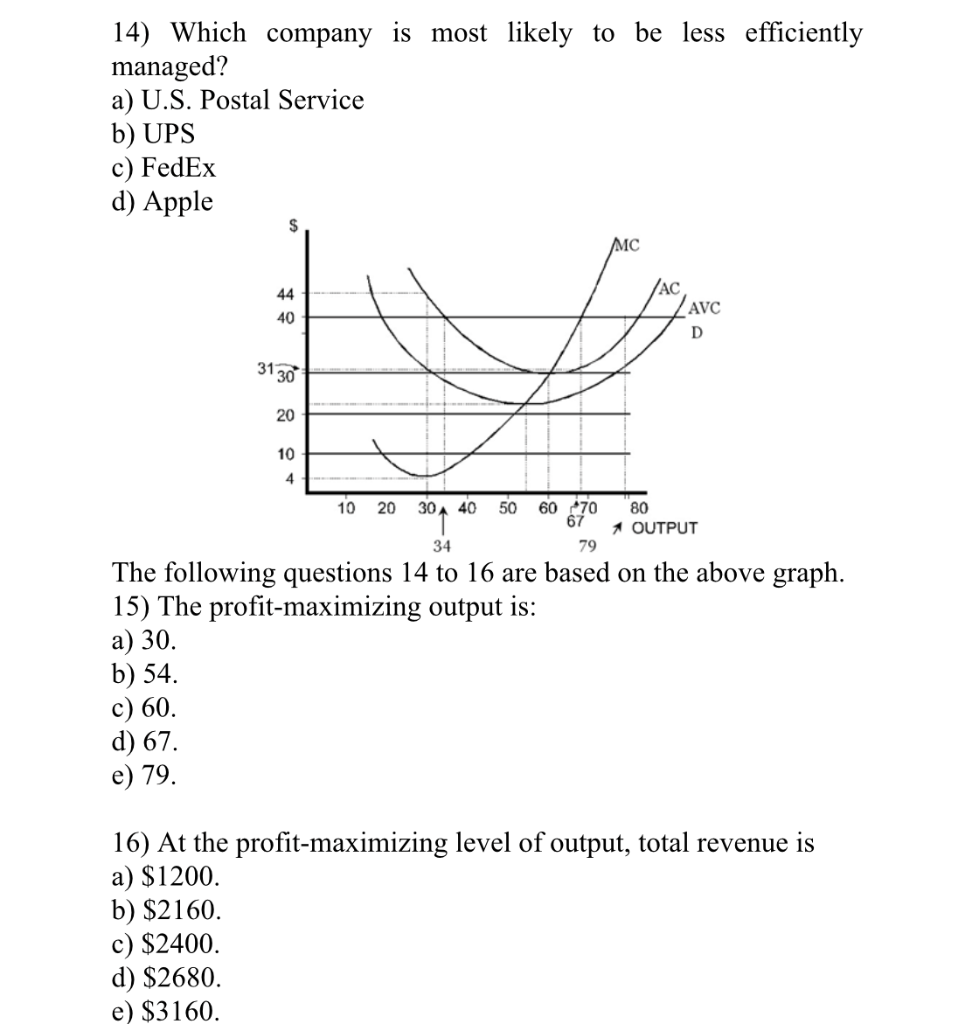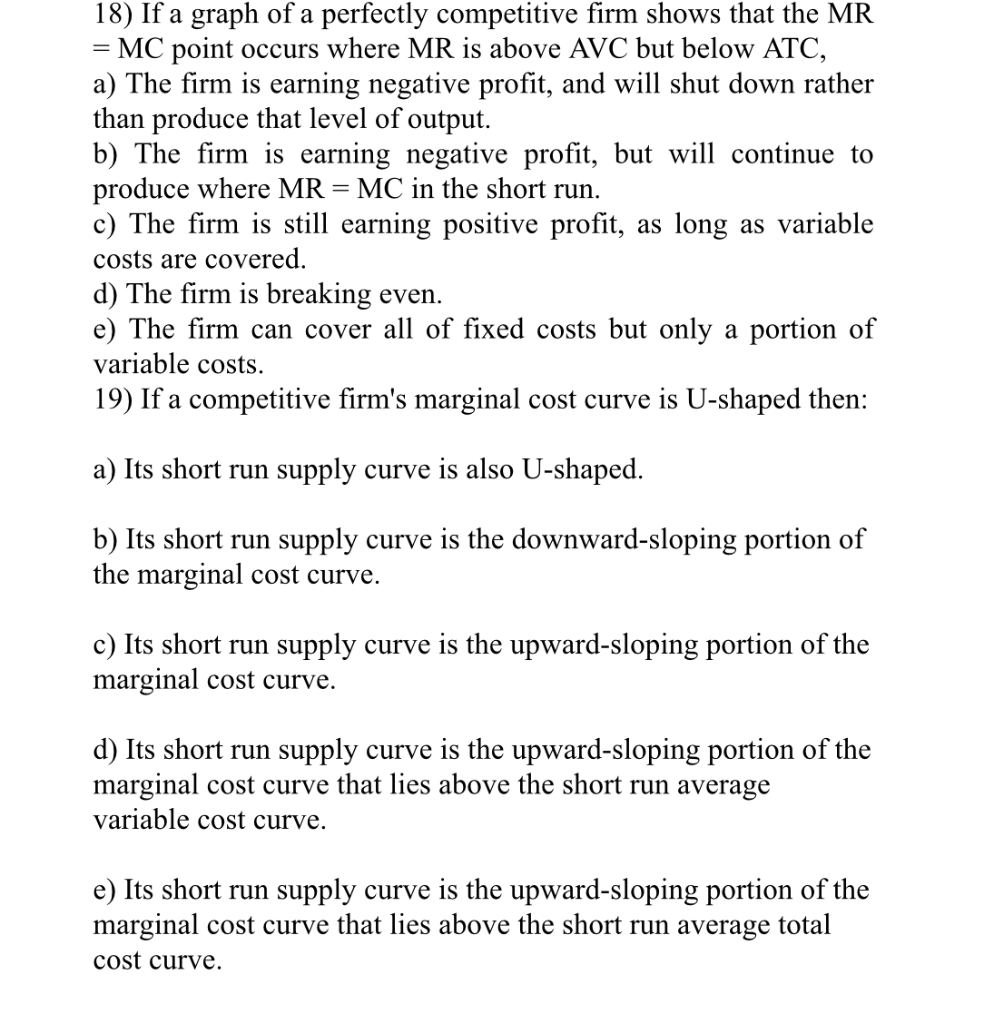Homework Help Question & Answers

14) Which company is most likely to be less efficiently managed? a) U.S. Postal Service b) UPS c)...

Please answer all multiple choices . i would be very thankful14) Which company is most likely to be less efficiently managed? a) U.S. Postal Service b) UPS c) FedEx d) AppleS IC AC AVC 30 20 4 10 20 30A 40 50 60 70 80 67 OUTPUT 34 79 The following questions 14 to 16 are based on the above graph 15) The profit-maximizing output is: a) 30 b) 54. c) 60 d) 67 e) 79 16) At the profit-maximizing level of output, total revenue is a) \$1200 b) \$2160 c) \$2400 d) \$2680. e) \$3160
18) If a graph of a perfectly competitive firm shows that the MR - MC point occurs where MR is above AVC but below ATO, a) The firm is earning negative profit, and will shut down rather than produce that level of output. b) The firm is earning negative profit, but will continue to produce where MR MC in the short run c) The firm is still earning positive profit, as long as variable costs are covered d) The firm is breaking even e) The firm can cover all of fixed costs but only a portion of variable costs. 19) If a competitive firm's marginal cost curve is U-shaped then: a) Its short run supply curve is also U-shaped b) Its short run supply curve is the downward-sloping portion of the marginal cost curve, c) Its short run supply curve is the upward-sloping portion of the marginal cost curve,. d) Its short run supply curve is the upward-sloping portion of the marginal cost curve that lies above the short run average variable cost curve. e) Its short run supply curve is the upward-sloping portion of the marginal cost curve that lies above the short run average total cost curve.

a) "D"

Apple will be less efficiently managed because they have a monopoly in the production of the MacBooks and other product. these are the products that only Apple can produce giving them a monopoly in the market.

b) "D"

The firm will produce 69 units because, that is the point where the MC and price are equal .

c) total revenue = \$40 x 67 = \$2680. The answer is "D".

d) "D"

Te firm is making a negative profit but will continue to produce in the short run, it should be above the short run average variable cost.

e)   "C"

The supply curve will be the upward sloping part of the MC curve.

Add Answer of: 14) Which company is most likely to be less efficiently managed? a) U.S. Postal Service b) UPS c)...
More Homework Help Questions Additional questions in this topic.

• company A:1/5 company B:4/25 company C:2/5 company D:3/20 how much more than company A's fraction of the market did company C have

Need Online Homework Help?

Get FREE EXPERT Answers
WITHIN MINUTES
Related Questions